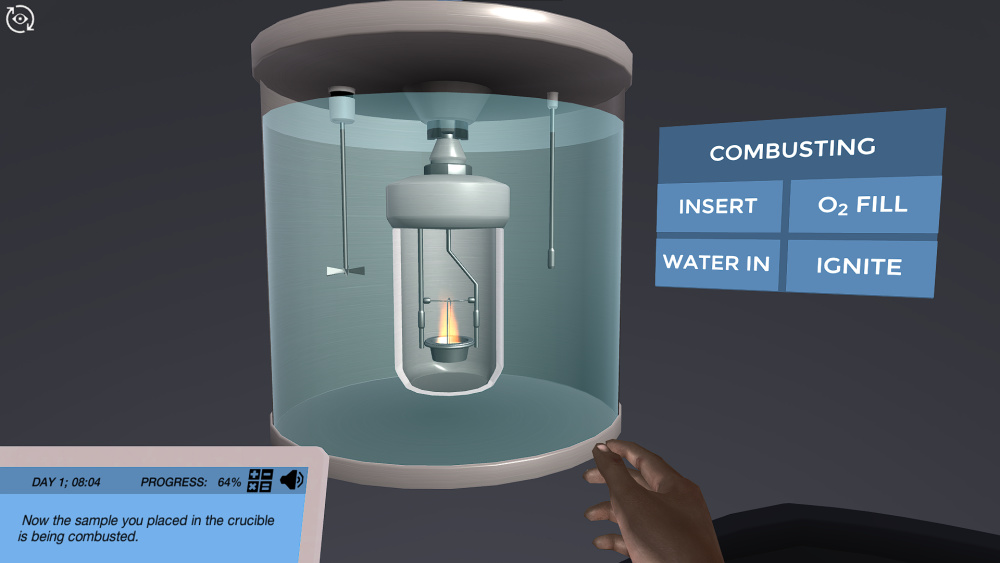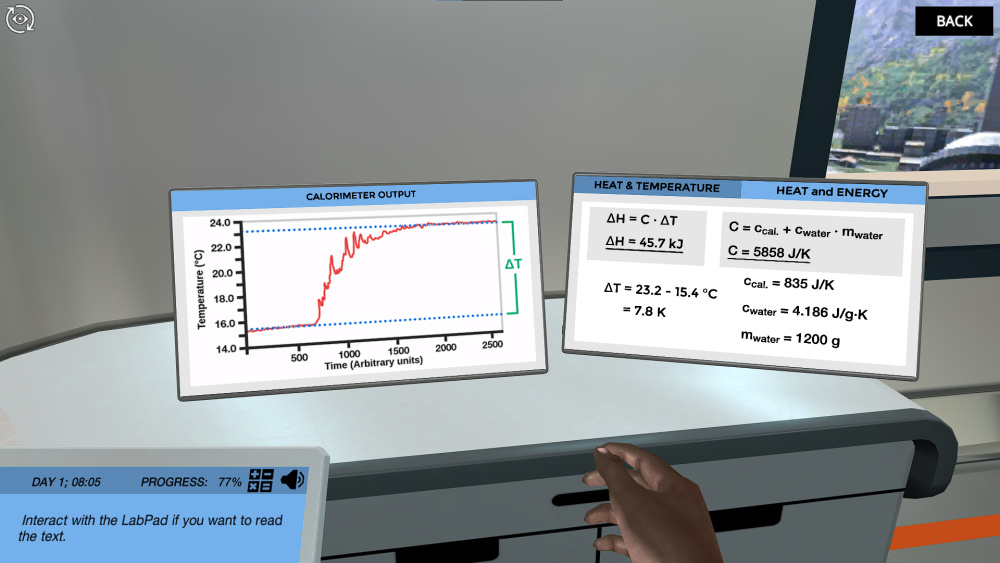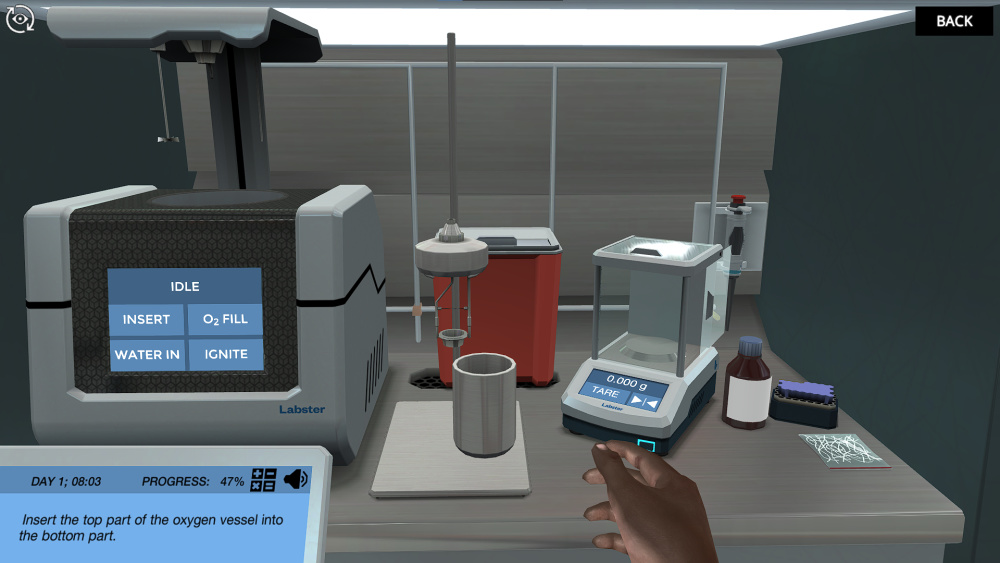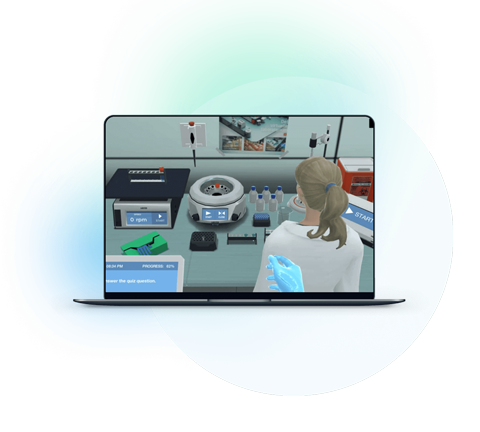Blog

# 5 Ways to Make Calorimetry and the Bomb Calorimeter More Approachable

Usama Attiq
Teaching with Labster
October 11, 2022

## Introduction: Calorimetry

Before we start the calorimetry, students should know the principle of the first law of thermodynamics. The first law of thermodynamics states that energy cannot be formed or destroyed but it can transform from one type to another type. So, it means that the energy of the world always remains constant. The principle of calorimetry indicates the first law of thermodynamics.

Calorimetry is a technique that can be used to measure the amount of heat involved in a chemical reaction. In this technique, you can measure the amount of energy that can transform from one substance to another substance. A calorimeter is a device that is used to measure the change of heat energy in a chemical reaction. When the temperature of the reaction medium changes, it shows the output of the calorimeter.

There are two types of calorimeters; constant volume calorimeter and constant pressure calorimeter. A constant volume calorimeter is also referred as a bomb calorimeter. It is used to measure heat change in a combustion reaction. A constant pressure calorimeter is mainly used to measure the heat in a liquid medium.

The explanation of the calorimeter and using a bomb calorimeter make some teachers and students feel overwhelmed.  Read on to know why this topic is difficult and learn five ways to understand calorimetry. In the end, we will be able to convince you why a lab simulation is important for students as well as teachers to better understand a tough topic like calorimetry.

## Why calorimetry can be tricky to learn

There are some reasons that explain why a calorimeter and using a bomb calorimeter are difficult for students to study.

### 1. It feels abstract

Sometimes, students find it difficult to learn about bomb calorimeters because they do not see the device and the process. When they learn the theory about the bomb calorimeter, it may be boring for them. The students do not imagine the working or picture of a bomb calorimeter after reading from the book.

### 2. It’s content-heavy

Calorimetry is a complex topic that consists of different types of calorimeters with several equations and applications. Each type of calorimeter has different working, construction, and purpose. There are several terms that students need to be remembered to get knowledge about calorimetry. Sometimes, students may be confused and find it tough to understand the calorimetry.

### 3. Complicated formulas

A bomb calorimeter consists of several formulas that are used to measure the energy involved in the reaction. Students need to convert the equations to calculate the desired value. Therefore, it may be hard for some students to remember the formulas and conversion of equations. Without using these equations, a student cannot calculate the exact values of heat capacity, change in temperature, or total heat capacity.

## 5 ways to make calorimetry a more approachable topic to understand

Since you know the main reasons that make calorimeters and using a bomb calorimeter a difficult topic, here are some ways that make calorimetry an interesting topic to read.

### 1. Talk about the people behind the bomb calorimeter

To explain the calorimetry and use of bomb calorimeter to the students, it is important to tell them about the person behind the successful invention. It enhances the knowledge of students about the scientist that work to make a bomb calorimeter.

Pierre Eugene Berthelot (1827-1907) was a great scientist who invented the bomb calorimeter. He was interested in developing different techniques in the field of thermochemistry. Berthelot wanted to create a device that measured the heat absorbed and resealed during a chemical reaction. He made a device that comprised a strong container and paced a sample with food or fuel. Then, the container was sealed after adding an excess of oxygen and igniting it. However, Berthelot’s bomb calorimeter can measure the amount of heat more accurately as compared with previous methods.

Berthelot also introduced the term “exothermic” and “endothermic”. Exothermic is a process in which heat is released from the reaction. While endothermic is a process in which heat is absorbed in the reaction.

### 2. Learn about Bomb Calorimeter

A bomb calorimeter is a device that is used to measure the heat of combustion in the reaction at a constant volume. It is also called a constant volume calorimeter. The device is designed to isolate the inner material from the surroundings, which means that no heat can enter or leave the system. Basically, the bomb calorimeter consists of a sample, stainless steel bomb, water, and oxygen. The outer wall of the bomb calorimeter vacuum seal. The reaction is in a wall inside the calorimeter and is surrounded by a copper vessel containing a water bath.

When you perform a chemical reaction in a bomb calorimeter, the temperature of the water bath changes.  A thermometer can be used to measure the temperature of the water bath. The temperature of the water indicates whether a reaction is exothermic or endothermic. If an endothermic reaction occurs in the bomb calorimeter, the temperature of the water bath becomes down. If an endothermic reaction takes place in the bomb calorimeter, the temperature of the water bath is raised.

There are some important terms that should know about calorimetry.

Enthalpy: Enthalpy refers to the heat of the system. Temperature and heat are two different terms but they can be related with a simple formula.

Total heat capacity: In a bomb calorimeter, the total heat capacity is used to compare changes in heat and changes in temperature.

Specific heat capacity: Specific heat capacity is defined as the amount of heat needed to raise the temperature of 1g of a substance by one degree. For example, the value of the specific heat capacity of water is 4.2 J/g degree.Figure: An image shows the inside view of bomb calorimeter from Labster’s virtual laboratory of calorimetry using a bomb calorimeter.

### 3. Use color diagrams

Students can understand a science topic like bob calorimeter when they see the device or experiment in person. A bomb calorimeter is a device, and reactions are taking place within it. The color images help students to understand the topic in a better way. When students see color diagrams of graphs and equations of a bomb calorimeter, the diagram will save in their minds. So, learning through dolor diagrams and images are effective to remember complex topic like bomb calorimeter.

The color image, like the one below, can help to understand the graph of the calorimeter, the formula of heat and temperature, and the formula of heat and energy. It can help the students to learn the formula and the values of specific constants more accurately.Figure: An image of calorimeter output graph and formulas from Labster's virtual lab calorimeter using a bomb calorimeter

### 4. Explains the uses of bomb calorimeter

Bomb calorimeter has several uses as it can tell us whether certain products are safe to use or not. Here are some important uses of the bomb calorimeter.

• Bomb calorimeter for fuel testing: The calorific value of fuel is tested through a bomb calorimeter. This specific value is used for trading purposes. In this process, the amount of energy of the fuel is determined by the heat of combustion.

• Education training: Bomb calorimeter is used for educational training purposes. In high schools and university levels, calorimetry is taught to the student.  They can perform practicals by using a bomb calorimeter. The practical use of the bomb calorimeter helps the student to understand the process effectively.

• Hazardous waste removal: Some industries like the cement industry use hazardous waste products instead of fuel. The government allows the use of hazardous waste products. A bomb calorimeter is used to determine that the hazardous waste product meets the required regulations and is safe for use in industries.

• Determination of calorie content of the products: Another use of the bomb calorimeter is to determine the calorie content of the products. In metabolic studies, a bomb calorimeter is used to check the effects of energy present in food on humans and animals.

• To learn thermodynamics: a Bomb calorimeter is used to learn about the processes of thermodynamics. It helps to measure the heat of combustion of a chemical reaction. Bomb calorimeters also measure the change in enthalpy, the heat involved in the reaction, and reaction enthalpy.

### 5. Use of virtual lab simulation

A virtual lab simulation is a great way to teach the students about the bomb calorimeter. At Labster, we provide you an advanced lab stimulation that consists of gamification elements such as a scoring system and storytelling to provide complete detail about the topic.

Labster’ssimulation for calorimetry using a bomb calorimeter explains the basic steps and critical points of a calorimeter, the first law of thermodynamics, the relation between enthalpy and internal energy, and the basic terms of thermodynamics. The virtual lab makes things simpler to understand for students as well as teachers.Check out the simulation for calorimetry using a bomb calorimeter, or get in touch to find out how you can start using virtual labs with your students.What exactly are virtual labs?